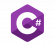# Difference between revisions of "CreateObject"

Creates a world object.

# C# Syntax`Object NAPI.Object.CreateObject(int model, Vector3 pos, Quaternion rot, uint dimension = NAPI.GlobalDimension);`
`Object NAPI.Object.CreateObject(uint model, Vector3 pos, Quaternion rot, uint dimension = NAPI.GlobalDimension);`
`Object NAPI.Object.CreateObject(int model, Vector3 pos, Vector3 rot, byte alpha = 255, uint dimension = NAPI.GlobalDimension);`
`Object NAPI.Object.CreateObject(uint model, Vector3 pos, Vector3 rot, byte alpha = 255, uint dimension = NAPI.GlobalDimension);`

### Parameters

• model: The model of the object to be created.
• pos: The position where the object will be created.
• rot: The rotation applied to the object.
• alpha: Optional, makes the object have transparency.
• dimension: Optional, the dimension from which the object will be seen.

### Example

`// Code here...`